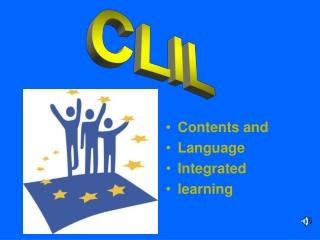DownloadDownload PresentationContents and Language Integrated learning

# Contents and Language Integrated learning

Download Presentation## Contents and Language Integrated learning

- - - - - - - - - - - - - - - - - - - - - - - - - - - E N D - - - - - - - - - - - - - - - - - - - - - - - - - - -
##### Presentation Transcript

1. CLIL • Contents and • Language • Integrated • learning

2. Plane Geometry Congruence, equivalence and similarity

3. congruence

4. Congruence • Two figures are congruent if they have exactly the same size and shape.

5. Precisely two plane figures are said congruent, if there is a rigid motion (which does not lead to deformation), which can lead one of the two figures to coincide with another .

6. Congruence • When two figures are congruent, there is a correspondence between their angles and sides such that corresponding angles are congruent and corresponding sides are congruent.

7. equivalence

8. equivalence • In geometry plane, equivalence is the propriety of two or more plane figures which have the same extension.

9. equivalence • The equivalence is a relationship between geometric figures that do not necessarily have the same shape, but have the same area.

10. equivalence • These figures are equivalent because they are equally composed

11. equivalence • The equivalence relation has three properties which are reflexive, symmetric and transitive.

12. reflexive propriety • a figure is always equivalent to itself

13. symmetric • if the figure a is equivalent to b, then b is equivalent to a;

14. transitive • Finally, if a is equivalent to b and b is equivalent to c, then a is equivalent to c A B C

15. similarity

16. similarity These two postcards, which are similar, aren`t the same This feature of polygons is calledsimilarity

17. similarity There is similarity between two or more polygons if all corresponding angles are congruent and all corresponding sides are proportional.

18. similarity Figures that have the same shape, but do not have the same size, have a relationship of similarity A B

19. BY NICK AND VICTOR